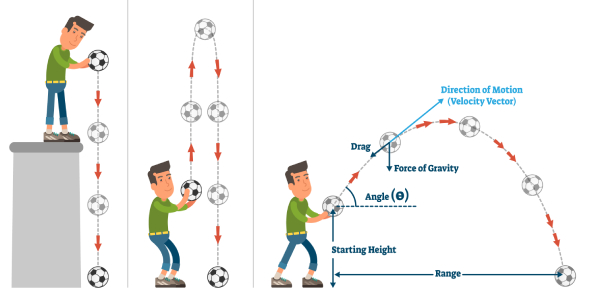# Projectile Motion Quiz Questions With Answers

15 Questions | Attempts: 7068
ShareSettingsDid you like studying projectile motion in physics? Try out these projectile motion quiz questions with answers, and see how much you know. This assessment will become a part of the body of evidence used to determine your grade. In other words, doing well can improve your grade, and doing poorly can lower your grade. All the best for a perfect score on this quiz. If you find the quiz informative and helpful, do share it with others who are interested in practicing projectile motion.

• 1.
A stone with an initial velocity of zero is dropped from a bridge above a river. After 3 s, the stone strikes the water below the bridge. Approximately how fast is the stone traveling when it strikes the water?
• A.

10 m/s

• B.

20 m/s

• C.

30 m/s

• D.

45 m/s

• 2.
A ball is projected vertically upwards with an initial velocity of 50 m.s-1. Using the formula 'v = u + at,' the calculated velocity after 2s is?
• A.

-30.4 m.s-1

• B.

30.4 m.s-1

• C.

-69.6 m.s-1

• D.

69.6 m.s-1

• 3.
The diagram below shows a football being kicked. Angle θ represents the angle between the horizontal and the ball's initial direction of motion. Which value of θ would result in the ball traveling the longest distance?
• A.

25 degrees

• B.

45 degrees

• C.

60 degrees

• D.

90 degrees

• 4.
If a golf ball is hit with more force at the same angle, its initial acceleration will
• A.

Decrease

• B.

Increase

• C.

Remain the same

• 5.
A pitching machine launches a softball horizontally with no spin. Which of the following statements correctly describes the ball’s motion in the air if the machine is set so that the launch speed is increased?
• A.

The ball’s velocity increases, and the distance it falls in one-second decreases

• B.

The ball’s velocity remains the same, and the distance the ball falls in one-second decreases

• C.

The ball’s velocity increases and the distance the ball falls in one-second increases

• D.

The ball’s velocity increases and the distance the ball falls in one-second remains the same

• 6.
A punter on the football team can kick the ball at an angle of either 30° or 80°. If he wants to maximize the distance the ball travels, at which angle should he kick the ball?
• A.

The 30° angle because the ball goes further

• B.

The 80° angle because the ball goes further

• C.

The 30° angle because the ball spends more time in the air

• D.

The 80° angle because the ball spends more time in the air

• 7.
A soccer ball is kicked so that at the peak of its trajectory, it has a horizontal speed of 21 m/s, and is 6 meters above the ground. The entire flight takes 2.2 seconds.  How far away from the kicker does the soccer ball land?
• A.

21 meters

• B.

6 meters

• C.

13.2 meters

• D.

46.2 meters

• 8.
A diver jumps horizontally off a cliff with an initial velocity of 6.0 m/s. The diver strikes the water 3.5 s later. a) What is the approximate vertical speed of the diver upon reaching the surface of the water? b) What is the horizontal speed of the diver 2.5 seconds after the diver jumps? c) How far from the base of the cliff will the diver strike the water?
• 9.
Two objects are released from the same height. Object A falls straight down from the cliff, and object B follows a curved parabolic path. Which of these is true?
• A.

A hits the ground before B

• B.

B hits the ground before A

• C.

A hits the ground first only if it is heavier than B

• D.

A and B hit the ground at the same time.

• 10.
Projectile motion has two components, horizontal (x) and vertical (y) as can be seen below. The horizontal motion has:
• A.

Constant acceleration

• B.

Changing acceleration

• C.

Constant velocity

• D.

Changing velocity

• E.

Both velocity and acceleration

• 11.
A cannon fires a 1kg cannonball with a velocity of 100m/s at 60 degrees to the horizontal and from a cliff which is 20m above sea level. What is the vertical component of its velocity?
• A.

50m/s

• B.

86.6m/s

• C.

90 m/s

• D.

100m/s

• 12.
A cannon fires a 1kg cannonball with a velocity of 100m/s at 60 degrees to the horizontal and from a cliff which is 20m above sea level How high will it reach?
• A.

127m

• B.

254m

• C.

382m

• D.

Option 4

• 13.
When a ball is projected horizontally, what is its initial vertical velocity?
• A.

0

• B.

8

• C.

10

• D.

Insufficient information

• 14.
What force acts in the y direction that causes an object to travel in a parabolic arc?
• A.

Tension

• B.

Friction

• C.

Gravity

• D.

No force

• 15.
A man wants to shoot an arrow so that it has the greatest range (horizontal distance). At what angle should he shoot the arrow?
• A.

45 degrees

• B.

60 degrees

• C.

90 degrees

• D.

120 degrees

## Related TopicsBack to top
×

Wait!
Here's an interesting quiz for you.